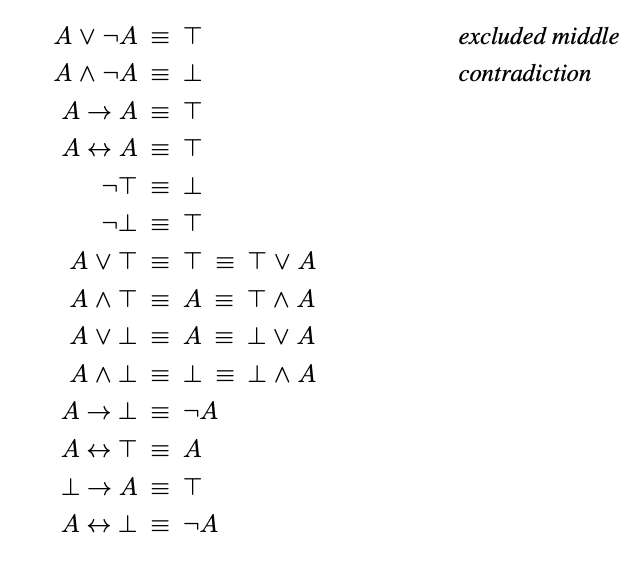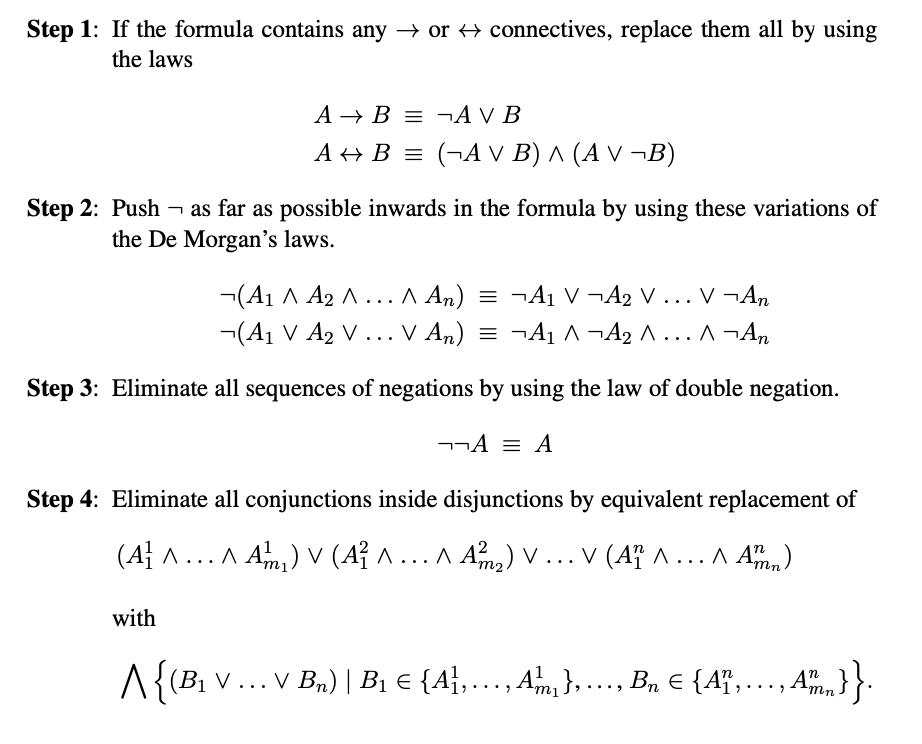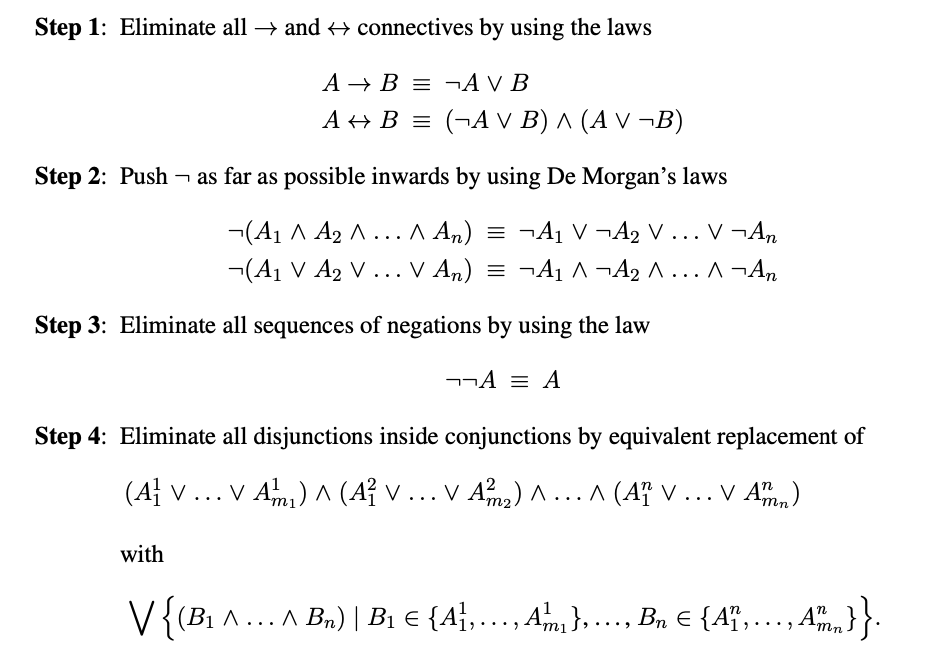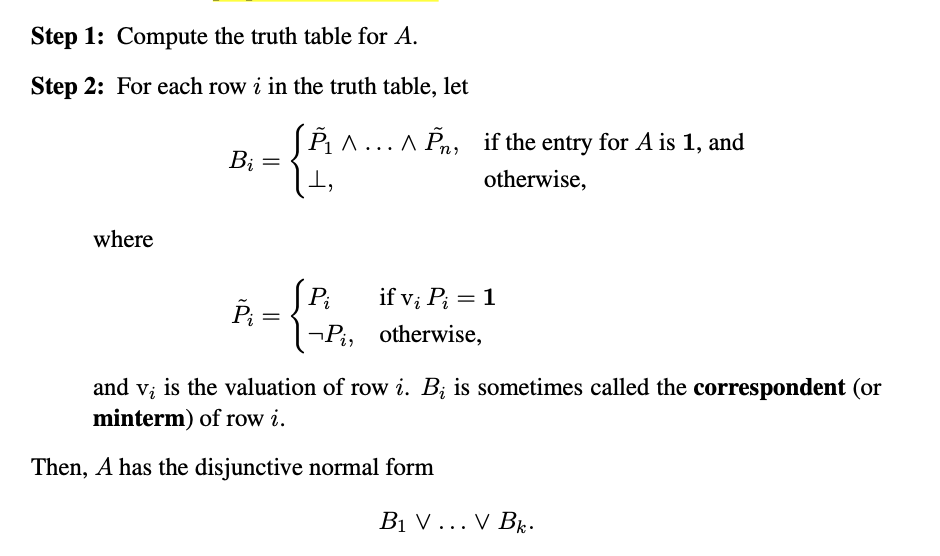# 逻辑学探幽 Part1

## 没有逻辑, 只有heip

Posted by R1NG on November 16, 2020 Viewed Times

# 逻辑表达式的等价定义和正规化

## 1. 逻辑表达式的等价

### 1.1 语义等价 (Semantic Equivalence)

1. 特别地, 在布尔语义下, 若两个谓词公式共享同一个真值表, 即可称其语义学等价.
2. 若 $A \equiv B$, 我们也称 $A$ 和 $B$ 逻辑意义上相等.
3. 在布尔语义下使用真值表法判断两个谓词公式语义学等价时, 真值表內必须包含组成这两个谓词公式的所有子式!
4. 通过使用真值表法, 不难得知: $\neg A \vee B \equiv A \rightarrow B.$

### 1.2 命题变量的替代

$\neg \neg P \equiv P$ 双否定性
$P \wedge P \equiv P$ 幂等性
$P \vee P \equiv P$ 幂等性
$P \wedge Q \equiv Q \wedge P$ 交换律
$P \vee Q \equiv Q \vee P$ 交换律
$P \wedge (Q \wedge R) \equiv (P \wedge Q) \wedge R$ 结合律
$P \vee (Q \vee R) \equiv (P \vee Q) \vee R$ 结合律
$P \wedge (Q \vee R) \equiv (P \wedge Q)\vee(P \wedge R)$ 分配律
$P \vee (Q \wedge R) \equiv (P \vee Q)\wedge(P \vee R)$ 分配律
$P \wedge (P \vee Q) \equiv P$ 吸收性
$P \vee (P \wedge Q) \equiv P$ 吸收性
$\neg(P \wedge Q) \equiv \neg P \vee \neg Q$ De Morgan
$\neg(P \vee Q) \equiv \neg P \wedge \neg Q$ De Morgan
$P \rightarrow Q \equiv \neg P \vee Q$ 逆否性
$P \rightarrow Q \equiv \neg(P \wedge \neg Q)$ 逆否性
$P \rightarrow Q \equiv \neg Q \rightarrow \neg P$ 逆否性
$P \leftrightarrow Q \equiv (P \rightarrow Q) \wedge (Q \rightarrow P)$ 逆否性
$P \leftrightarrow Q \equiv (\neg P \vee Q) \wedge (P \vee \neg Q)$ 逆否性
$P \leftrightarrow Q \equiv (P \wedge Q) \vee (\neg P \wedge \neg Q)$ 逆否性

$A \equiv B$ 当且仅当 $A \leftrightarrow B$ 为重言式.

$A(P_1, P_2, \cdots, P_n) \equiv B(P_1, P_2, \cdots, P_n)$

$A(C_1, C_2, \cdots, C_n) \equiv B(C_1, C_2, \cdots, C_n).$

$\neg \neg A \equiv A$ 双否定性
$A \wedge A \equiv A$ 幂等性
$A \vee A \equiv A$ 幂等性
$A \wedge B \equiv B \wedge A$ 交换律
$A \vee B \equiv B \vee A$ 交换律
$A \wedge (B \wedge R) \equiv (A \wedge B) \wedge R$ 结合律
$A \vee (B \vee R) \equiv (A \vee B) \vee R$ 结合律
$A \wedge (B \vee R) \equiv (A \wedge B)\vee(A \wedge R)$ 分配律
$A \vee (B \wedge R) \equiv (A \vee B)\wedge(A \vee R)$ 分配律
$A \wedge (A \vee B) \equiv A$ 吸收性
$A \vee (A \wedge B) \equiv A$ 吸收性
$\neg(A \wedge B) \equiv \neg A \vee \neg B$ De Morgan
$\neg(A \vee B) \equiv \neg A \wedge \neg B$ De Morgan
$A \rightarrow B \equiv \neg A \vee B$ 逆否性
$A \rightarrow B \equiv \neg(A \wedge \neg B)$ 逆否性
$A \rightarrow B \equiv \neg B \rightarrow \neg A$ 逆否性
$A \leftrightarrow B \equiv (A \rightarrow B) \wedge (B \rightarrow A)$ 逆否性
$A \leftrightarrow B \equiv (\neg A \vee B) \wedge (A \vee \neg B)$ 逆否性
$A \leftrightarrow B \equiv (A \wedge B) \vee (\neg A \wedge \neg B)$ 逆否性

### 1.3 命题变量的等价代换

$C(\cdots A \cdots)$

$C(\cdots A \cdots) \equiv C(\cdots B \cdots)$

### 1.4 逻辑常量 $\perp$, $\top$

$P$ $\neg P$ $P \vee \neg P$ $P \wedge \neg P$
$1$ $0$ $1$ $0$
$0$ $1$ $0$ $1$## 2. 正规形式和逻辑表达式的正规化

$P_1\wedge (P_2 \wedge (\cdots \wedge P_n)\cdots) \Rightarrow P_1\wedge P_2 \wedge \cdots \wedge P_n.$

### 2.1 合取范式 (Conjunctive Normal Form)• 合取范式求解算法的每一步都保持语义学等价关系.
• 合取范式求解算法总会在有限步內终止.
• 合取范式求解算法的返回值恒为一个合取范式.

### 2.2 析取范式 (Disjunctive Normal Form)• 析取范式求解算法的每一步都保持语义学等价关系.
• 析取范式求解算法总会在有限步內终止.
• 析取范式求解算法的返回值恒为一个析取范式.

### 2.3 基于真值表构造范式### 2.4 布尔函数